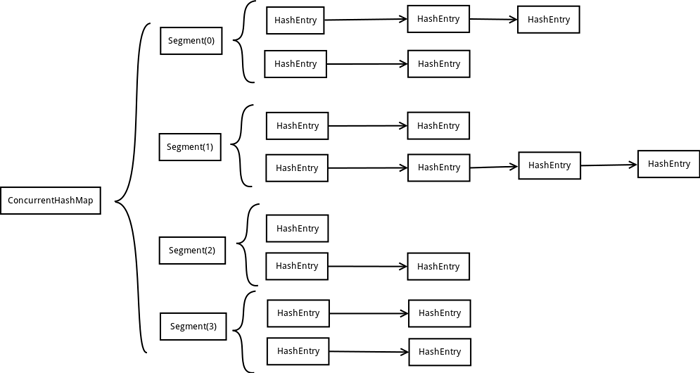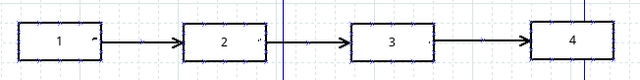求知 文章 文库 Lib 视频 iPerson 课程 认证 咨询 工具 讲座 Modeler Code分享到
Java并发编程之ConcurrentHashMap

ConcurrentHashMap

ConcurrentHashMap是一个线程安全的Hash Table，它的主要功能是提供了一组和HashTable功能相同但是线程安全的方法。ConcurrentHashMap可以做到读取数据不加锁，并且其内部的结构可以让其在进行写操作的时候能够将锁的粒度保持地尽量地小，不用对整个ConcurrentHashMap加锁。

ConcurrentHashMap的内部结构

ConcurrentHashMap为了提高本身的并发能力，在内部采用了一个叫做Segment的结构，一个Segment其实就是一个类Hash Table的结构，Segment内部维护了一个链表数组，我们用下面这一幅图来看下ConcurrentHashMap的内部结构：Segment

 ``` static final class Segment extends ReentrantLock implements Serializable { transient volatile int count; transient int modCount; transient int threshold; transient volatile HashEntry[] table; final float loadFactor; } ```

• count：Segment中元素的数量
• modCount：对table的大小造成影响的操作的数量（比如put或者remove操作）
• threshold：阈值，Segment里面元素的数量超过这个值依旧就会对Segment进行扩容
• table：链表数组，数组中的每一个元素代表了一个链表的头部

HashEntry

Segment中的元素是以HashEntry的形式存放在链表数组中的，看一下HashEntry的结构：

 ```static final class HashEntry { final K key; final int hash; volatile V value; final HashEntry next; }```

ConcurrentHashMap的初始化

 ```public ConcurrentHashMap(int initialCapacity, float loadFactor, int concurrencyLevel) { if (!(loadFactor > 0) || initialCapacity < 0 || concurrencyLevel <= 0) throw new IllegalArgumentException(); if (concurrencyLevel > MAX_SEGMENTS) concurrencyLevel = MAX_SEGMENTS; // Find power-of-two sizes best matching arguments int sshift = 0; int ssize = 1; while (ssize < concurrencyLevel) { ++sshift; ssize <<= 1; } segmentShift = 32 - sshift; segmentMask = ssize - 1; this.segments = Segment.newArray(ssize); if (initialCapacity > MAXIMUM_CAPACITY) initialCapacity = MAXIMUM_CAPACITY; int c = initialCapacity / ssize; if (c * ssize < initialCapacity) ++c; int cap = 1; while (cap < c) cap <<= 1; for (int i = 0; i < this.segments.length; ++i) this.segments[i] = new Segment(cap, loadFactor); } ```

ConcurrentHashMap的get操作

 ```public V get(Object key) { int hash = hash(key.hashCode()); return segmentFor(hash).get(key, hash); } ```

 ```final Segment segmentFor(int hash) { return segments[(hash >>> segmentShift) & segmentMask]; } ```

 ```V get(Object key, int hash) { if (count != 0) { // read-volatile HashEntry e = getFirst(hash); while (e != null) { if (e.hash == hash && key.equals(e.key)) { V v = e.value; if (v != null) return v; return readValueUnderLock(e); // recheck } e = e.next; } } return null; } ```

 ` transient volatile int count; `

 ```HashEntry getFirst(int hash) { HashEntry[] tab = table; return tab[hash & (tab.length - 1)]; } ```

ConcurrentHashMap的put操作

 ```V put(K key, int hash, V value, boolean onlyIfAbsent) { lock(); try { int c = count; if (c++ > threshold) // ensure capacity rehash(); HashEntry[] tab = table; int index = hash & (tab.length - 1); HashEntry first = tab[index]; HashEntry e = first; while (e != null && (e.hash != hash || !key.equals(e.key))) e = e.next; V oldValue; if (e != null) { oldValue = e.value; if (!onlyIfAbsent) e.value = value; } else { oldValue = null; ++modCount; tab[index] = new HashEntry(key, hash, first, value); count = c; // write-volatile } return oldValue; } finally { unlock(); } } ```

ConcurrentHashMap的remove操作

Remove操作的前面一部分和前面的get和put操作一样，都是定位Segment的过程，然后再调用Segment的remove方法：

 ```V remove(Object key, int hash, Object value) { lock(); try { int c = count - 1; HashEntry[] tab = table; int index = hash & (tab.length - 1); HashEntry first = tab[index]; HashEntry e = first; while (e != null && (e.hash != hash || !key.equals(e.key))) e = e.next; V oldValue = null; if (e != null) { V v = e.value; if (value == null || value.equals(v)) { oldValue = v; // All entries following removed node can stay // in list, but all preceding ones need to be // cloned. ++modCount; HashEntry newFirst = e.next; for (HashEntry p = first; p != e; p = p.next) newFirst = new HashEntry(p.key, p.hash, newFirst, p.value); tab[index] = newFirst; count = c; // write-volatile } } return oldValue; } finally { unlock(); } } ```ConcurrentHashMap的size操作

 分享到

 Java 中的中文编码问题 Java基础知识的三十个经典问答 玩转 Java Web 应用开发 使用Spring更好地处理Struts 用Eclipse开发iPhone Web应用 插件系统框架分析

 Struts+Spring+Hibernate 基于J2EE的Web 2.0应用开发 J2EE设计模式和性能调优 Java EE 5企业级架构设计 Java单元测试方法与技术 Java编程方法与技术

 Struts+Spring+Hibernate/EJB+性能优化 华夏基金 ActiveMQ 原理与管理 某民航公司 Java基础编程到应用开发 某风电公司 Java 应用开发平台与迁移 日照港 J2EE应用开发技术框架与实践 某跨国公司 工作流管理JBPM 东方航空公司 高级J2EE及其前沿技术